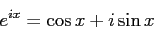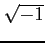Euler's formula, named after Leonhard Euler, is a mathematical formula in that shows a deep relationship between the trigonometric functions and the complex exponential function. Euler's formula states that, for any real number x,where

e
is the base of the natural logarithm
i
is the imaginary unit, i.e.cos and sin
are the trigonometric functions cosine and sine respectively
x
is the arguement, given in radians

The formula is still valid if x is a complex number.

Richard Feynman called Euler's formula "our jewel"[Feynman, Richard P. (1977). The Feynman Lectures on Physics, vol. I.Addison-Wesley.] and "one of the most remarkable, almost astounding, formulas in all of mathematics".

danny 2009-08-22